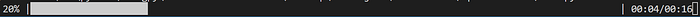# How to Create a Progress Bar in Python

## How to use the tqdm library to easily add progress bars to your command line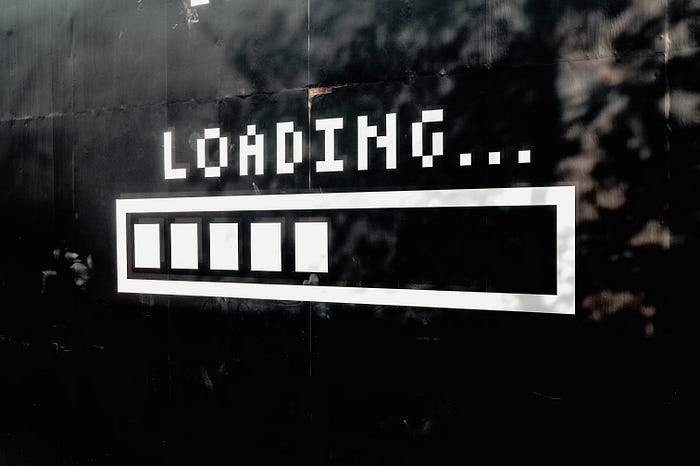In this guide, I will show you how to easily add progress bars to your command line. This is particularly useful if you are working on a Machine Learning project, where the program is based on a loop that can take some time to execute.

In general, a progress bar can make it easier to understand the progress that your program is making and how long it will take, whenever it is based on a loop that is repeated many times.

### Tqdm: basic usage

Tqdm is a great library, that allows you to add really easily a progress bar to your code.

First of all, let’s install this library:

``````pip install tqdm
``````

Then, to simulate a for loop that takes some time to execute, we will use the `time.sleep` method:

``````import time
for i in range(10):
time.sleep(2)
``````

If you execute this code, each iteration will take approximately two seconds, but there is no way to know while it is executing at what point it is.

We can solve this by using the `tqdm` object of the tqdm library:

``````from tqdm import tqdm
import time
for i in tqdm(range(10)):
time.sleep(2)
``````

Now, when you execute the code, this will be displayed in your command line: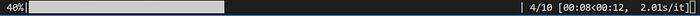On the left, it shows the percentage that has been completed. On the right, it shows the number of the current iteration and the total number of iterations, as well as the time taken so far and the expected remaining time. The last number is the approximate time needed per iteration.

#### The trange object

You will often need the command `tqdm(range(...))` . Instead, you can use `trange` , which works in the same way. SO we could rewrite our program as:

``````from tqdm import trange
import time
for i in trange(10):
time.sleep(2)
``````

Now that we have seen the basic usage of this library, we will explore some advanced parameters of the `tqdm` class.

#### Description

First of all, we can add a description to our progress bar. This can be particularly useful if you have multiple loops, each with a different bar, and you want to distinguish between them.

To do this, you can use the `desc` parameter:

``````from tqdm import tqdm
import time
bar = tqdm(range(10), desc="Example bar")
for i in bar:
time.sleep(2)
``````

This description will be shown on the left side of the bar: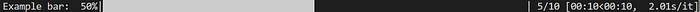#### Custom Stats

If you want to implement some custom stats to show in the progress bar, you will have to use the `set_postfix` function to update it at each iteration. This function will take as a parameter a dictionary, with all the stats that should be shown.

Here is an example of this:

``````from tqdm import tqdm
import time
bar = tqdm(range(10))tot = 0
for i in bar:
time.sleep(2)
tot += i
bar.set_postfix({"total":tot})
``````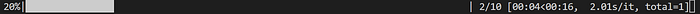#### Format

To customize the format of the progress bar, you can also use the `bar_format` parameter. This takes an f-string as input, which can contain the following elements:

• `bar` the progress bar
• `desc` the description
• `n_fmt` the current iteration number
• `total_fmt` the total number of iterations
• `percentage` the percentage that has been executed so far
• `elapsed` the time elapsed from the beginning of the loop
• `remaining` the expected remaining time
• `rate_fmt` the time for iteration
• `postfix` the stats added by `set_postfix`

For example, this is a simple format that only shows the percentage (with no decimal digits) and the time elapsed and remaining:

``````from tqdm import tqdm
import timebar=tqdm(range(10),
bar_format="{percentage:3.0f}% |{bar}| {elapsed}/{remaining}"
)
for i in bar:
time.sleep(2)
``````